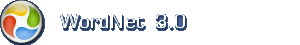# Engelsk ordbog

Tip: Jokertegn må gerne anvendes flere gange i hver søgning.

## Engelsk navneord: geometry

1. geometry (om erkendelse) the pure mathematics of points and lines and curves and surfaces

Mindre specifikke termerpure mathematics

Mere specifikke termeraffine geometry, analytic geometry, analytical geometry, coordinate geometry, descriptive geometry, elementary geometry, Euclidean geometry, fractal geometry, non-Euclidean geometry, parabolic geometry, plane geometry, projective geometry, solid geometry, spherical geometry

Overordnet emneområdemath, mathematics, maths

Indenfor samme emneområdeangle of inclination, circumscribe, congruent, conic, conic section, construct, diagonal, duality, eccentricity, foursquare, inclination, incongruent, inscribe, normal, pencil, plane section, right, section, square, superposition, translate, truncate

Baseret på WordNet 3.0 copyright © Princeton University.
Teknik og design: Orcapia v/Per Bang. Dansk bearbejdning: .
2019 onlineordbog.dk